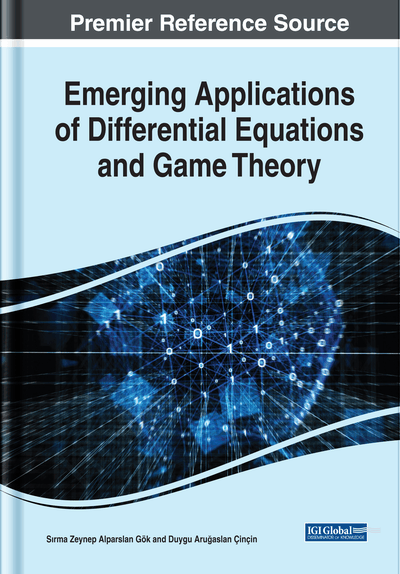# A Generalization of Groups: Partial Groups

Zekiye Çiloğlu Şahin (Süleyman Demirel University, Turkey) and Yılmaz Çeven (Süleyman Demirel University, Turkey)
DOI: 10.4018/978-1-7998-0134-4.ch001
Available
\$37.50
No Current Special Offers

## Abstract

For a nonempty set G, the authors define an operation * reckoned with closeness property (i.e.,* is an operation which is not a binary operation). Then they define the partial group as a generalisation of a group. A partial group G which is a non empty set satisfies following conditions hold for all a,b and c∈d:(PG1) If ab,(ab)c, bc and a (bc) is defined, then,(ab) c=a (bc)(PG2) . For every,a∈G there exists an e∈G such that ae and ea are defined and, ae=ea=a (PG3) . For every,a∈G there exists an a∈G such that aa and aa are defined and aa=aa=e. The * operation effects and changes the properties of group axioms. So that lots of group theoretic theorems and conclusions do not work in partial groups. Thus, this description gives us some fundemental and important properties and analogous to group theory. Also the authors have some differences from group theory.
Chapter Preview
Top

## Introduction

Groupoid is an algebraic structure which is first introduced by Brandt in “Über eine Verallgemeinerung des Gruppengriffes”. The definition of a groupoid is given as the following:

Let G be a nonempty set. A binary operation on G is a mapping from G×G to G. If * is a binary operation on G, then it is known that a*bG for all a,bG. (*(a,b) is denoted by a*b).

A partially defined binary operation on G is a function from D to G i.e. *:DG, where D is a subset of G×G.

If an operation * is a partially defined binary operation on G, then a*b is undefined or a*b is not contained in G for some a,bG.

A semigroupoid (which is also called as partial semigroup by Bergelson V., Blass A. and Hindman N., in “Partition theorems for spaces of variable words”) is a nonempty set G equipped with a partially defined binary operation * on G and this operation is associative in the following sense:

If either (a*b)*c or a*(b*c) is defined, then so is the other and.

Let (G,*) is a semigroupoid. Then G is called a groupoid if it satisfies the following conditions:

An algebraic structure called as an effect algebra, has recently been introduced for investigations in the foundations of quantum mechanics by Foulis D., and Bennett M. K., in the paper “Effect algebras and unsharp quantum logics”.

Moreover, effect algebras play a fundamental role in recent investigations of fuzzy probability theory. The connection between effect algebras and probablity theory can be found in “Fundamentals of fuzzy probability theory” by Bugajski S.

The definition of an effect algebra is given as the following:

An effect algebra is an algebraic system (E,0,1,*) where 0,1 are distinct elements of E and * is a partially defined binary operation on E that satisfies the following conditions for all a,b and cE:

## Complete Chapter List

Search this Book:
Reset